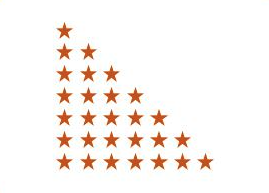# C Program to Print Right Triangle Star Pattern

Here is a C program to print a right angles triangle pattern of star (*) character using loops. For a right triangle star pattern of 7 rows. Program's output should be:#### Required Knowledge

Algorithm to print right triangle star pattern using loop
• Take the number of rows(N) of right triangle as input from user using scanf function.
• Number of stars in Kth row is always K. 1st row contains 1 star, 2nd row contains 2 stars, 3rd row contains 3 stars. In general, Kth row contains K stars.
• We will use two for loops to print right triangle star pattern.
• For a right triangle star pattern of N rows, outer for loop will iterate N time. Each iteration of outer loop will print one row of the pattern.
• For Kth row of right triangle pattern, inner loop will iterate K times. Each iteration of inner loop will print one star (*).

Here is the matrix representation of the triangle star pattern. The row numbers are represented by i whereas column numbers are represented by j.## C program to print right triangle star pattern

```#include<stdio.h>

int main() {
int i,j,rows;
printf("Enter the number of rows\n");
scanf("%d", &rows);

for(i = 0; i < rows; i++) {
/* Prints one row of triangle */
for(j = 0; j <= i; ++j) {
printf("* ");
}
/* move to next row */
printf("\n");
}
return 0;
}
```
Output
```Enter the number of rows
6
*
* *
* * *
* * * *
* * * * *
* * * * * *
```

Related Topics
 C program binary triangle pattern C program palindrome triangle pattern C program natural number triangle pattern C program pyramid star pattern C program diamond star pattern C program inverted right triangle pattern C program heart shape star pattern C program mirrored right triangle star pattern C program exponentially increasing star pattern List of all C pattern programs